数据结构和算法难？盘他！- 快速入门Aldeo定义算法的效率

空间对效率的影响-空间复杂度

for 循环

public static void main(String[] args) {        int a = 10000;        for (int i=1;i<=a;i++) {            System.out.println(i);        }    }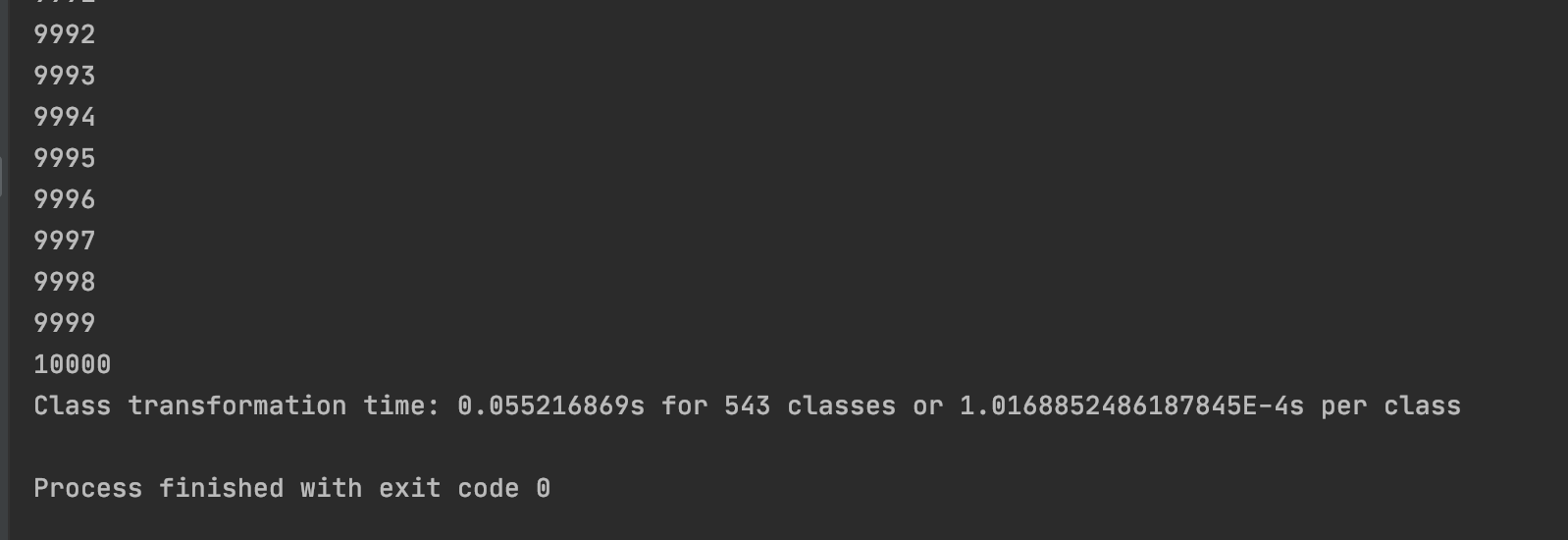递归

public static void main(String[] args) {        int a = 10000;        aa(a);    }
static void aa(int a){ a = a - 1; if (a > 0 ) { System.out.println(a); aa(a); } }

478547844783Class transformation time: 0.069511503s for 543 classes or 1.2801381767955802E-4s per class*** java.lang.instrument ASSERTION FAILED ***: "!errorOutstanding" with message transform method call failed at JPLISAgent.c line: 844*** java.lang.instrument ASSERTION FAILED ***: "!errorOutstanding" with message transform method call failed at JPLISAgent.c line: 844Exception in thread "main" java.lang.StackOverflowError

• 递归深度为 N*每次递归的辅助空间大小，如果每次递归的辅助空间为常数，则空间复杂度为 O(N)。

• 对于递归的二分查找，递归深度是 log2^n，每次递归的辅助空间为常数，所以空间复杂度为 O(log2N)（2 为底数下标）

算法的巧妙程度对效率的影响-时间复杂度

计算：多项式的值

方法一：直接计算

public static void main(String[] args) {        double[] a = new double[]{1,2,3};        System.out.println(f1(2,a,3));        System.out.println(f2(2,a,3));    }
//不思考，直接堆堆方法 static double f1(int n,double a[],double x){ long start = System.currentTimeMillis(); int i; double p = a; for (i = 1;i <= n;i++){ p += a[i] * Math.pow(x, i); } long end = System.currentTimeMillis(); //计算执行时间 System.out.println(end - start + "ms"); return p; }
//使用公式堆方法 static double f2(int n,double a[],double x){ long start = System.currentTimeMillis(); int i; double p = a[n]; for (i = n;i > 0;i--){ p = a[i-1] + x*p; } long end = System.currentTimeMillis(); //计算执行时间 System.out.println(end - start + "ms"); return p; }

两种方法的执行效率

public static void main(String[] args) {        double[] a = new double[]{1,2,3};        f1(2, a, 3);        f2(2, a, 3);    }
//不思考，直接堆堆方法 static void f1(int n,double a[],double x){ long start = System.currentTimeMillis(); //重复执行10的7次方次 double flag = Math.pow(10, 7); for (int k = 1;k<=flag;k++) { int i; double p = a; for (i = 1;i <= n;i++){ p += a[i] * Math.pow(x, i); } } long end = System.currentTimeMillis(); //计算执行时间 System.out.println((end - start)/flag + "ms"); }
//使用公式堆方法 static void f2(int n,double a[],double x){ long start = System.currentTimeMillis(); //重复执行10的7次方次 double flag = Math.pow(10, 7); for (int k = 1;k<=flag;k++) { int i; double p = a[n]; for (i = n;i > 0;i--){ p = a[i-1] + x*p; } } long end = System.currentTimeMillis(); //计算执行时间 System.out.println((end - start)/flag + "ms"); }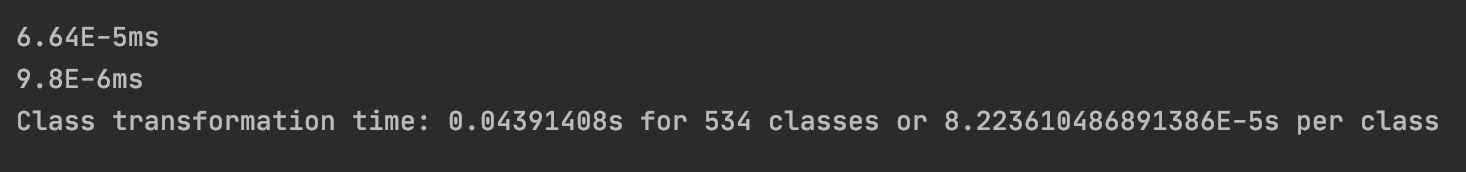时间复杂度的渐进表示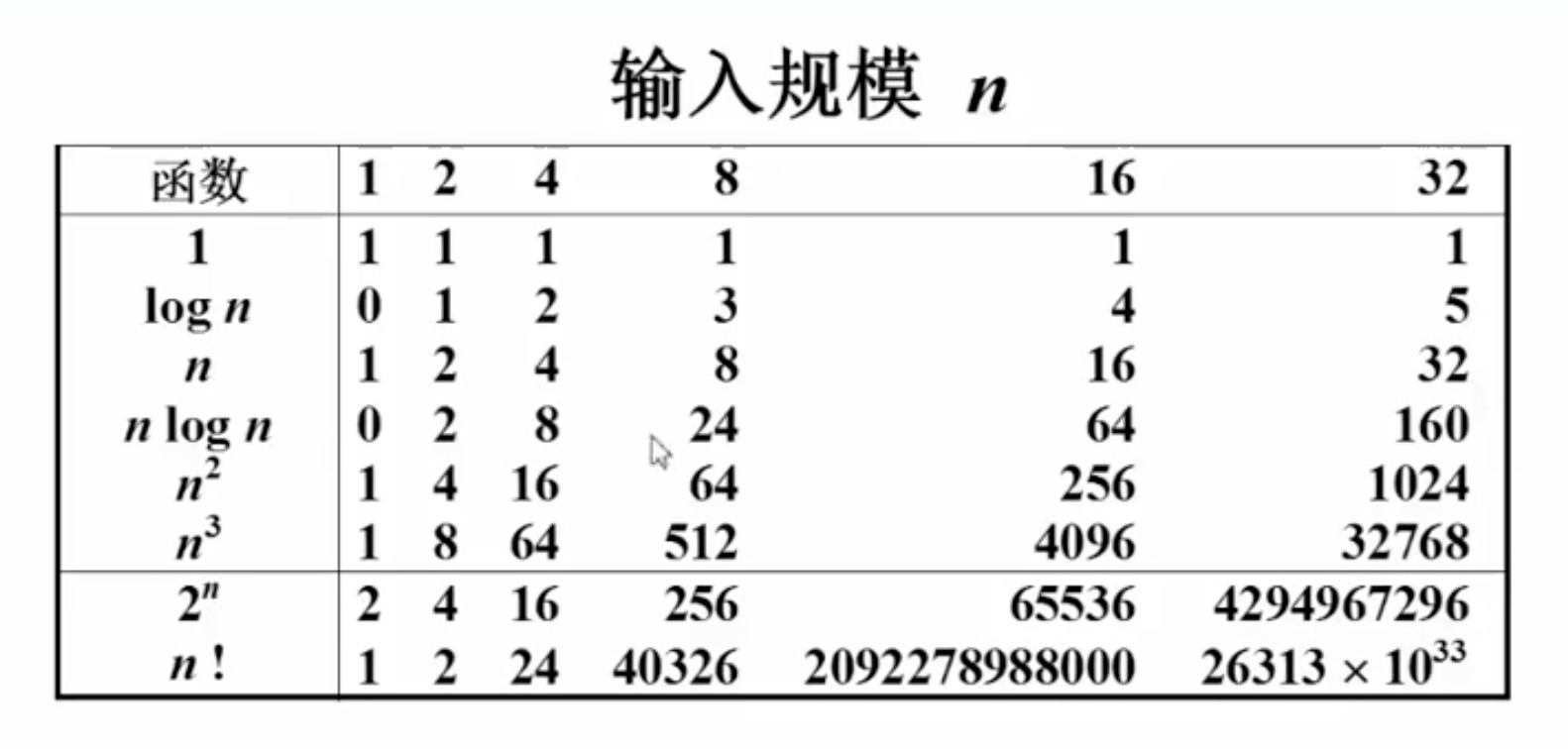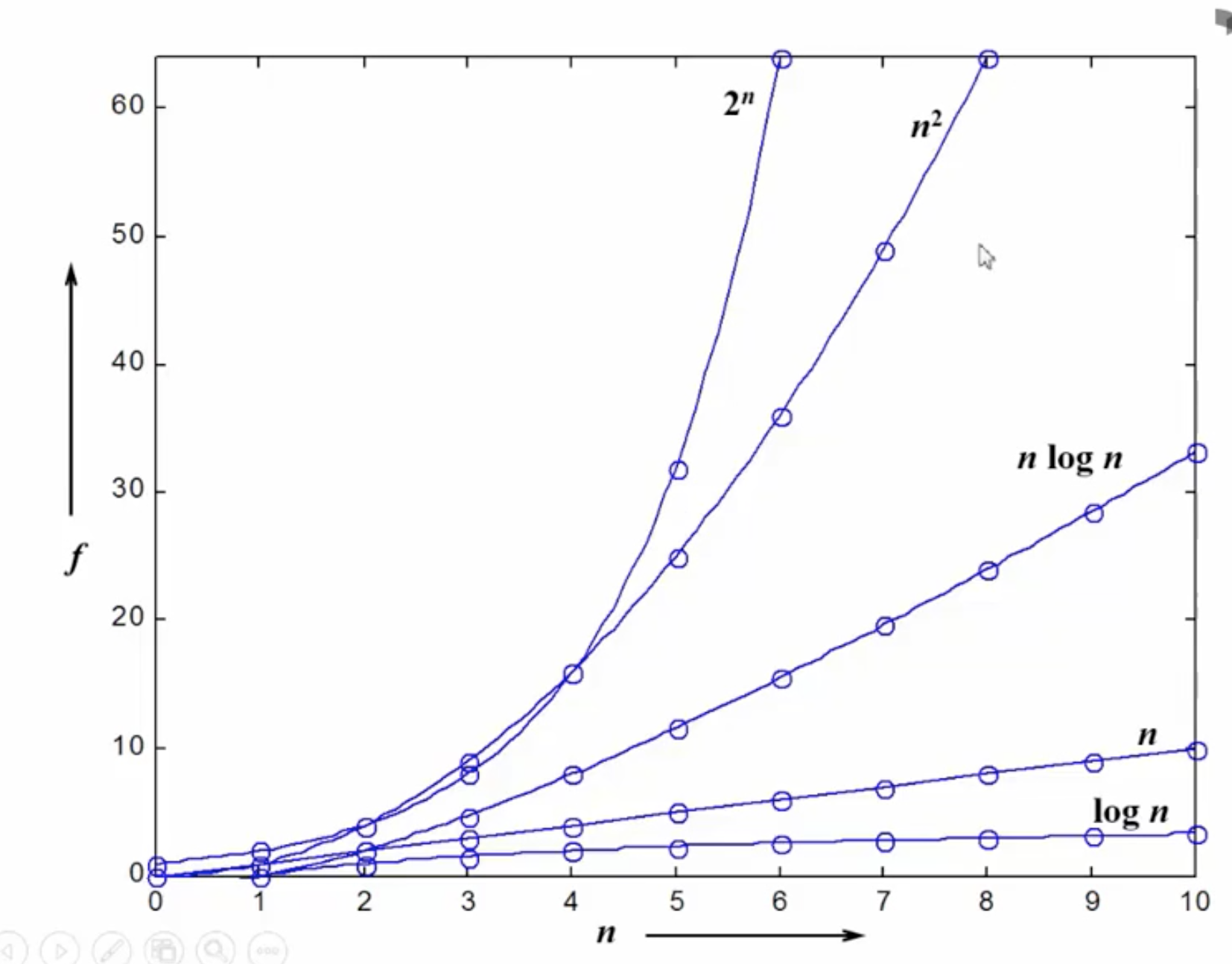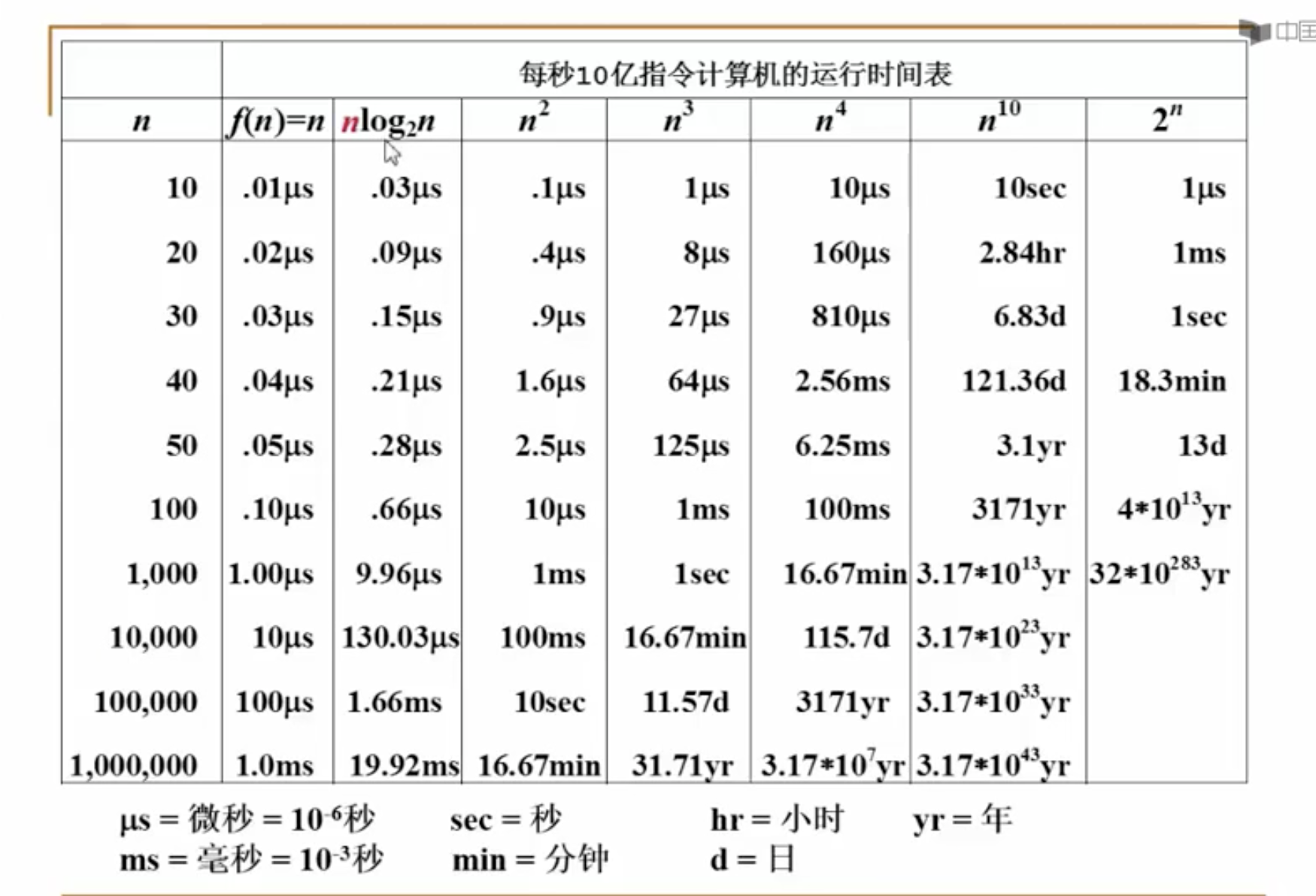小窍门

1.若两段算法的复杂度相加取最大算法的复杂度为其复杂度，即

2.若算法是关于 n 的 k 阶多项式，则复杂度为 n 的 k 阶

3.for 循环的复杂度=循环次数*循环体的复杂度

4.if-else 结构的复杂度=max（条件判断复杂度，if 代码块复杂度，else 代码块复杂度）

小结评论